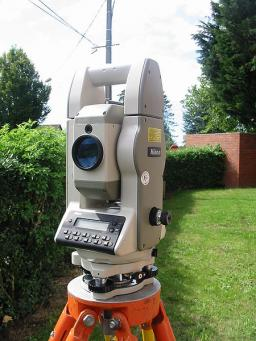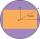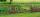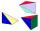# Surveyor

Calculate the area of ​​what may vary rectangular, if it focused by surveyor and found the dimensions 18 x 15 m while in each of the four joint points can be position deviation 25 cm?

Correct result:

ΔS =  23.3 m2

#### Solution:We would be pleased if you find an error in the word problem, spelling mistakes, or inaccuracies and send it to us. Thank you!Tips to related online calculators
Pythagorean theorem is the base for the right triangle calculator.

#### You need to know the following knowledge to solve this word math problem:

We encourage you to watch this tutorial video on this math problem:

## Next similar math problems:Calculate the surface of a quadrilateral pyramid, which has a rectangular base with dimensions a = 8 cm, b = 6 cm and height H = 10 cm.A quadrilateral pyramid, which has a rectangular base with dimensions of 24 cm, 13 cm. The height of the pyramid is 18cm. Calculate 1/the area of the base 2/casing area 3/pyramid surface 4/volume of the pyramid
• Again sawFrom the trunk of the tree we have to a sculpture beam with rectangular cross-section with dimensions 146 mm and 128 mm. What is the trunk smallest diameter?
• BillboardRectangular billboard is 2.5 m long with a diagonal 2.8 m long. Calculate the perimeter and the content area of the billboard.
• Rectangular base pyramidCalculate an area of the shell of the pyramid with a rectangular base of 2.8 m and 1.4 m and height 2.5 meters.
• AcreageWhat acreage has a rectangular plot whose diagonal is 34 meters long and one side has a length of 16 meters. ?
• Rectangular fieldA rectangular field has a diagonal of length 169m. If the length and width are in the ratio 12:5. Find the dimensions of the field, the perimeter of the field and the area of the field.
• The fieldThe player crossed the field diagonally and walked the length of 250 m. Calculate the length of the field, circumference if one side of field 25 meters.
• PagansElena cut out same circle-shaped pagans and put them on a rectangular sheet so that the neighboring pagans were touching each other and the pagans were touching the walls of the sheet on the edges. Each pagans occupied 28.26 cm2 of the bottom of the sheet
• Rectangular gardenThe sides of the rectangular garden are in ratio 1: 2. The diagonal has a length of 20 meters. Calculate the area and perimeter of the garden.
• Trapezoid MOThe rectangular trapezoid ABCD with right angle at point B, |AC| = 12, |CD| = 8, diagonals are perpendicular to each other. Calculate the perimeter and area of ​​the trapezoid.
• Diagonalhe rectangular ABCD trapeze, whose AD arm is perpendicular to the AB and CD bases, has area 15cm square. Bases have lengths AB = 6cm, CD = 4cm. Calculate the length of the AC diagonal.
• Two rectanglesI cut out two rectangles with 54 cm², 90 cm². Their sides are expressed in whole centimeters. If I put these rectangles together I get a rectangle with an area of 144 cm2. What dimensions can this large rectangle have? Write all options. Explain your calc
• Triangular prismCalculate the surface of a regular triangular prism, the edges of the base are 6 cm long and the height of the prism is 15 cm.
• Rectangular trapezoidCalculate the content of a rectangular trapezoid with a right angle at the point A and if |AC| = 4 cm, |BC| = 3 cm and the diagonal AC is perpendicular to the side BC.
• PyramidCuboid ABCDEFGH has dimensions AB 3 cm, BC 4 cm, CG 5 cm. Calculate the volume and surface area of a triangular pyramid ADEC.
• KiteJohn a kite, which is diamond shaped. Its diagonals are 60 cm long and 90 cm long. Calculate: a) the diamond side b) how much paper John needs to make a kite if he needs paper on both sides and needs 5% of the paper for bending.